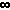Home    |    Teacher    |    Parents    |    Glossary    |    About UsEmail this page to a friendResources· Cool Tools · Formulas & Tables · References · Test Preparation · Study Tips · Wonders of MathSearchDerivatives: Min, Max, Critical Points... (Math | Calculus | Derivatives | Extrema/Concavity/Other)

Asymptotes

Definition of a horizontal asymptote: The line y = y0 is a "horizontal asymptote" of f(x) if and only if f(x) approaches y0 as x approaches + or -.

Definition of a vertical asymptote: The line x = x0 is a "vertical asymptote" of f(x) if and only if f(x) approaches + or -as x approaches x0 from the left or from the right.

Definition of a slant asymptote: the line y = ax + b is a "slant asymptote" of f(x) if and only if lim (x-->+/-) f(x) = ax + b.

Concavity
Definition of a concave up curve: f(x) is "concave up" at x0 if and only if f '(x) is increasing at x0

Definition of a concave down curve: f(x) is "concave down" at x0 if and only if f '(x) is decreasing at x0

The second derivative test: If f ''(x) exists at x0 and is positive, then f ''(x) is concave up at x0. If f ''(x0) exists and is negative, then f(x) is concave down at x0. If f ''(x) does not exist or is zero, then the test fails.

Critical Points
Definition of a critical point: a critical point on f(x) occurs at x0 if and only if either f '(x0) is zero or the derivative doesn't exist.
Extrema (Maxima and Minima)
Local (Relative) Extrema

Definition of a local maxima: A function f(x) has a local maximum at x0 if and only if there exists some interval I containing x0 such that f(x0) >= f(x) for all x in I.

Definition of a local minima: A function f(x) has a local minimum at x0 if and only if there exists some interval I containing x0 such that f(x0) <= f(x) for all x in I.

Occurrence of local extrema: All local extrema occur at critical points, but not all critical points occur at local extrema.

The first derivative test for local extrema: If f(x) is increasing (f '(x) > 0) for all x in some interval (a, x0] and f(x) is decreasing (f '(x) < 0) for all x in some interval [x0, b), then f(x) has a local maximum at x0. If f(x) is decreasing (f '(x) < 0) for all x in some interval (a, x0] and f(x) is increasing (f '(x) > 0) for all x in some interval [x0, b), then f(x) has a local minimum at x0.

The second derivative test for local extrema: If f '(x0) = 0 and f ''(x0) > 0, then f(x) has a local minimum at x0. If f '(x0) = 0 and f ''(x0) < 0, then f(x) has a local maximum at x0.

Absolute Extrema

Definition of absolute maxima: y0 is the "absolute maximum" of f(x) on I if and only if y0 >= f(x) for all x on I.

Definition of absolute minima: y0 is the "absolute minimum" of f(x) on I if and only if y0 <= f(x) for all x on I.

The extreme value theorem: If f(x) is continuous in a closed interval I, then f(x) has at least one absolute maximum and one absolute minimum in I.

Occurrence of absolute maxima: If f(x) is continuous in a closed interval I, then the absolute maximum of f(x) in I is the maximum value of f(x) on all local maxima and endpoints on I.

Occurrence of absolute minima: If f(x) is continuous in a closed interval I, then the absolute minimum of f(x) in I is the minimum value of f(x) on all local minima and endpoints on I.

Alternate method of finding extrema: If f(x) is continuous in a closed interval I, then the absolute extrema of f(x) in I occur at the critical points and/or at the endpoints of I.
(This is a less specific form of the above.)

Increasing/Decreasing Functions
Definition of an increasing function: A function f(x) is "increasing" at a point x0 if and only if there exists some interval I containing x0 such that f(x0) > f(x) for all x in I to the left of x0 and f(x0) < f(x) for all x in I to the right of x0.

Definition of a decreasing function: A function f(x) is "decreasing" at a point x0 if and only if there exists some interval I containing x0 such that f(x0) < f(x) for all x in I to the left of x0 and f(x0) > f(x) for all x in I to the right of x0.

The first derivative test: If f '(x0) exists and is positive, then f '(x) is increasing at x0. If f '(x) exists and is negative, then f(x) is decreasing at x0. If f '(x0) does not exist or is zero, then the test tells fails.

Inflection Points
Definition of an inflection point: An inflection point occurs on f(x) at x0 if and only if f(x) has a tangent line at x0 and there exists and interval I containing x0 such that f(x) is concave up on one side of x0 and concave down on the other side.